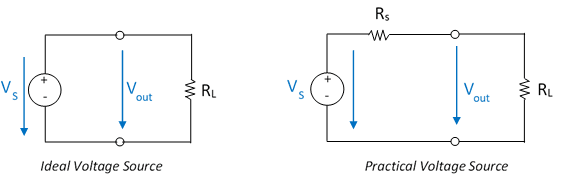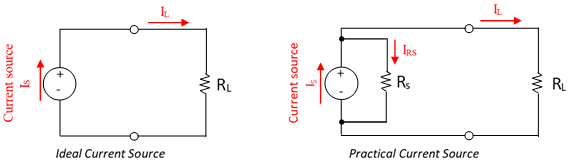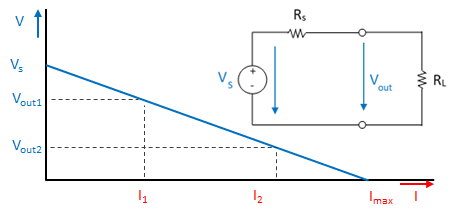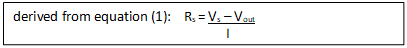# Practical Voltage and Current Sources, equivalent circuit diagram

## Practical Voltage and Current Sources, equivalent circuit diagram

### Voltage source

An ideal voltage source provides constant voltage at its terminal to the circuit regardless of the load current.

Practical voltage sources are real and used in daily life. A practical voltage source has internal resistance that causes a drop in terminal voltage due to the current flow.Ideal Voltage Source and Practical Voltage Source - equivalent circuit diagram

(Index S stands for source, L for load)

Note: The equivalent circuit diagram is a representation that can be used to calculate the true terminal voltage of a voltage source when current is drawn from it. Note that internal resistance is an inherent property of a source, it is not a discrete component that can be measured with an ohmmeter.

Regarding the Practical Voltage Source:

• If no load, the voltage at the terminals is the same as the internal voltage V0.
• With a load you get:  Vout = VS – RS * I
• In case of short circuit applies:  Imax = US / RS

### Current Sources

Current sources are active network elements that should provide the same current to any load connected across it.

Ideal current sources are providing the exact same current to any resistance connected to it. Where practical current sources may vary current resistance.Ideal Current Source and Practical Current Source and their equivalent circuit diagram

Regarding the Practical Current Source:

• With no load you get the voltage at the terminals:  V = Rs * I
• In case of short circuit applies: Imax = Iq

Let´s go into detail

### Characteristics of a Real Voltage Source

Due to the internal resistance Rs, the voltage at the terminals decreases with increasing current:Real voltage source - dependence of terminal voltage on load

The maximum terminal voltage can be obtained when no current is flowing. As the current increases, the terminal voltage decreases because a voltage drops across the internal resistor Rs:

Vout = Vs – VRs

Vout = Vs – Rs* I     … Eq. (1)

The short-circuit current is calculated as:     Imax = V0 / Rs

In the short-circuit case, i.e. when the terminals are short-circuited, the terminal voltage is of course 0V. However, this means that the total source voltage Vs drops across the internal resistor.

Determine the internal resistance:

It is not useful or often not possible to determine the short-circuit current. It is better to determine one point of the load characteristic curve, i.e. to connect a defined resistor to the terminals.

The second point can then be the case, when no load is connected to the terminals, that means I = 0A.If a current measurement is to be dispensed with, this equation can be transformed.

With I = Vout / RL you get: